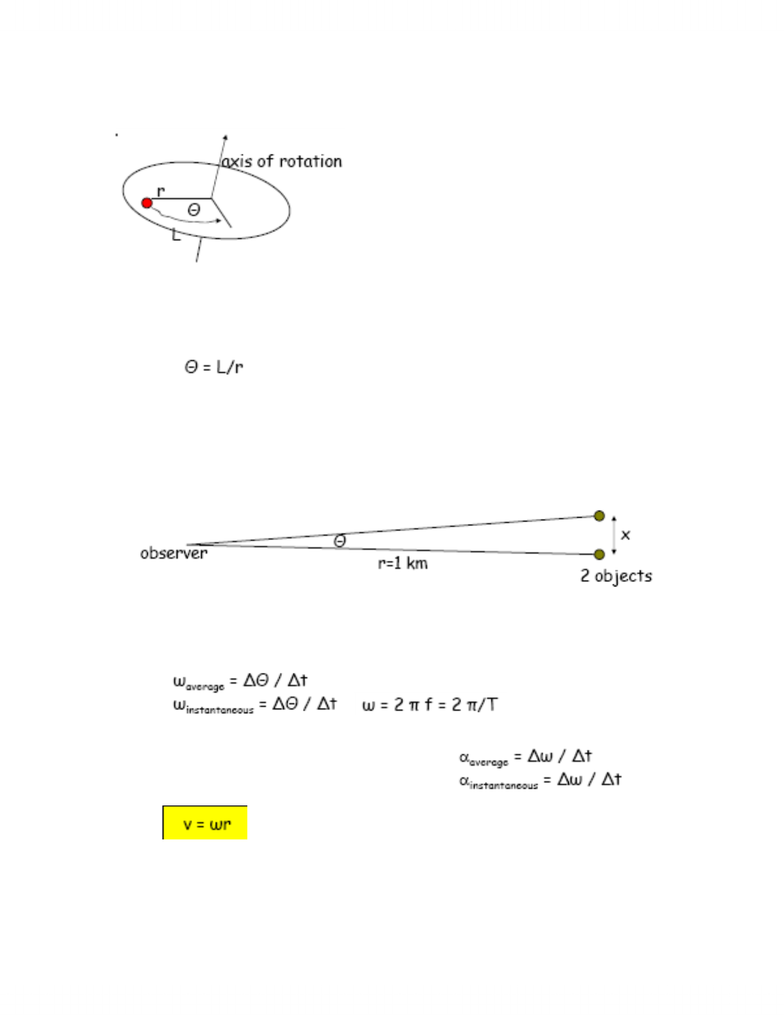Class Notes (1,100,000)
CA (620,000)
McGill (30,000)
Physics (500)
Lecture

# PHYS 101 Lecture Notes - Angular Acceleration, Angular Velocity, Angular Diameter

Department
Physics
Course Code
PHYS 101
Professor
Kenneth Ragan

This preview shows half of the first page. to view the full 3 pages of the document.Phys 101 Alanna Houston
October 23, 2007
Rotational Motion
-A point at a distance r moves through an arc of length L with: θ =
L/r
-Dealing mostly with radians!
-Objects with the same angular velocity do not always move at the
same speed.
-In , theta is in radians. Radians are useful when angles
are very small since we then have: sin(θ) = x/r = L/r = θ, sin(θ) =
θ, tan(θ) = θ
-The human eye can distinguish an object that subtends an angle
of ~ 5x10^-4 rads. What is the minimum spatial extent that can
be distinguished at 1 km distance? (To subtend an angle means to
have that angular size)
-
-small theta therefore theta is the ratio of x/r, x = 5x10^-4 * 1km
= 5m
-The angle theta is the equivalent of displacement x; the
equivalent of the velocity v is then the angular velocity ω.
-,
-All points on the rigid body rotate at the same rate ω or frequency
-Similarly, angular acceleration will be
-
-Example: A hard disk spins at 15000 rpm. If the disk has a
diameter of 3”, what is the linear velocity at the edge of the disk?
o1 inch = 2.54 cm, therefore r = 7.62/2 = 0.038 m
ov = ωr = (15000*2π/60) * 0.038 m = 60 m/s = 200 km/h
###### You're Reading a Preview

Unlock to view full version